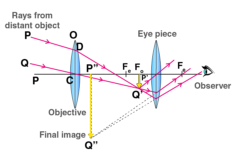# Draw a ray diagram of astronomical telescope for distance object in normal adjustment. What is the expression for its magnifying power.Ray diagram of an astronomical telescope with a normal adjustment is given below

The magnifying power is given by the formula

$$m = \frac{f_{0}}{f_{e}}$$

The final picture is at infinity in normal adjustment, to have high magnifying power fo must be as high as practically possible while fe should be kept small. The magnifying power is the minimum and its field of view is small.